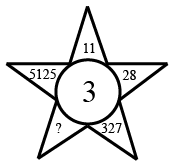Question 10

# Study the given pattern carefully and select the number that can replace the question mark (?) in itSolution

The logic here is

11 $$\longrightarrow\$$ 1$$^3$$ = 1

28 $$\longrightarrow\$$ 2$$^3$$ = 8

327 $$\longrightarrow\$$ 3$$^3$$ = 27

5125 $$\longrightarrow\$$ 5$$^3$$ = 125

Similarly, the missing number = 4..4$$^3$$ = 464

Hence, the correct answer is Option D

• Free SSC Study Material - 18000 Questions
• 230+ SSC previous papers with solutions PDF

##### Sharmi Ammu

3 years, 3 months ago

Explain....

##### Rehan Mohd

3 years, 1 month ago

Solution proper